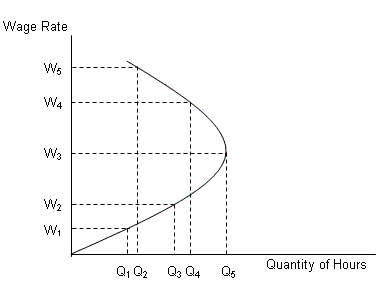/
/
/
MULTIPLE CHOICE 1.Which of the following a true statement about leisure? a.When
Not my Question
Flag Content

# Question : MULTIPLE CHOICE 1.Which of the following a true statement about leisure? a.When : 1413874

MULTIPLE CHOICE

1.Which of the following is a true statement about leisure?

a.When the salary of a low income earner increases, it often becomes possible to increase both labor and leisure.

b.Standing in line at the bank is not enjoyable and as a result is not considered leisure.

c.If someone enjoys his or her job, then the time he or she spends at work is considered leisure.

d.Leisure will always decrease as a person’s wage increases.

e.Leisure includes all hours that a person spends away from a paying job, regardless of how this time is spent.

2.Which of the following best explains the shape of the individual labor-supply curve?

a.The individual labor-supply curve is exactly like any supply curve, it always has a positive slope.

b.The individual labor-supply curve slopes downward at all wage rates because, as wages increase, people are able to buy more leisure.

c.The individual labor-supply curve slopes upward at lower wage rates and then bends back at higher wage rates.

d.The individual labor-supply curve must be vertical because each person can work only eight hours per day.

e.The individual labor-supply curve must be horizontal because labor markets are assumed to be perfectly competitive.

3.It is said that a wage increase can have two opposing effects. Which of the following captures these two effects?

a.A backward-bending labor-supply curve

b.A perfectly elastic labor-supply curve

c.A perfectly inelastic labor-supply curve

d.The inability of average wage earners to comprehend the complexities of economic principles.

e.A backward-bending labor-demand curve

4.Which of the following is a reason that some economists do not agree with the concept of a labor-leisure tradeoff?

a.Wages are paid in dollars and leisure is measured in time, hence there is no way to compare the two.

b.On a day-to-day basis, most jobs do not have the flexibility to allow people to weigh the benefits and costs to determine how much they should work that day.

c.In the long-run, the supply of labor hours is perfectly inelastic.

d.An increase in the wage rate always leads to an increase in the supply of labor hours.

e.Some people do not work at all, so there is no labor-leisure tradeoff for those individuals.

NARRBEGIN: Figure 15.1

The figure below shows the supply curve for labor.

Figure 15.1NARREND

5.Refer to Figure 15.1. When wage rate increases from W2 to W3 :

a.quantity of labor supplied increases from Q2 to Q4.

b.quantity of labor supplied decreases from Q3 to Q4.

c.quantity of labor supplied increases from Q4 to Q5.

d.quantity of labor supplied increases from Q2 to Q4.

e.quantity of labor supplied increases from Q3 to Q5.

6.Refer to Figure 15.1. When wage rate increases from W3 to W5:

a.quantity of labor supplied increases from Q1to Q4.

b.quantity of labor supplied decreases from Q5 to Q2.

c.quantity of labor supplied increases from Q2 to Q4.

d.quantity of labor supplied decreases from Q4 to Q2.

e.quantity of labor supplied increases from Q1 to Q54.

7.Which of the following statements contradicts the upward-sloping market-supply curve?`

a.All people have backward-bending individual supply curves

b.Leisure is more enjoyable than work

c.The elasticity of labor supply is larger than the elasticity of labor demand.

d.More work means less leisure

e.The opportunity cost of leisure decreases with an increase in wage rate.

8.Why is the market supply curve of labor positively sloped though individual supply curves are backward-bending beyond a certain wage rate?

a.People tend to work less when wage rate increases.

b.Every individual laborer has equal trade offs between labor and leisure.

c.People tend to substitute labor for leisure.

d.People work more for the fear of losing their jobs and becoming unemployed.

e.All the individual supply curves do not bend backward at one particular wage rate.

9.As the wage rate increases, the quantity supplied of labor in a market will:

a.invariably increase

b.invariably decrease.

c.first increase and then decrease.

d.first decrease and then increase.

e.remain constant.

10.The labor market is in equilibrium when:

a.the demand curve lies above the supply curve.

b.both demand and supply curves are positively sloped.

c.both demand and supply curves are negatively sloped.

d.the demand curve intersects the supply curve.

e.the demand curve is negatively sloped but the supply curve is positively sloped.

## Solution 5 (1 Ratings )

Solved
Economics 11 Months Ago 137 Views